#Function Repository Resource:

# HexStringToReal

Convert a hexadecimal string to a real number

Contributed by: Daniel Lichtblau
 ResourceFunction["HexStringToReal"][s] gives the machine double number corresponding to the hexadecimal string s, using 11 bits for the exponent. ResourceFunction["HexStringToReal"][s,{e,prec}] computes the real number in precision prec, taking e bits for the exponent field.

## Details and Options

When the hex string is converted to a list of binary bits, the first is used as the sign bit (0 for positive, 1 for negative).
After the sign bit and exponent bits are the mantissa bits.
The mantissa always begins just to the right of the radix, so the first bit is omitted since it must be 1 (often this is referred to as the “implicit” bit).
For machine floats with 64 bits, typical usage, reflected in the default settings of this function, is to have a sign bit, 11 exponent bits and 53 mantissa bits (one implicit and the next 52 explicit in the hexadecimal representation).
This function does not account for denormalized numbers, zeros or NaNs.

## Examples

### Basic Examples

Find the number for a hex‐encoded machine float:

 In:=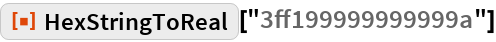Out=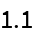Translate hex‐encoded string to a software float:

 In:=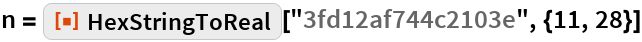Out=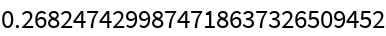Check that it is not a machine number:

 In:=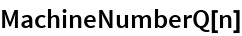Out=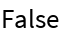The input form will also show that it has an associated precision:

 In:=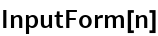Out=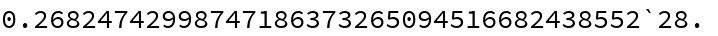### Possible Issues

Requesting more precision than is present in the hexadecimal representation will lead to padding with zero bits, which will appear as arbitrary digits in decimal form:

 In:=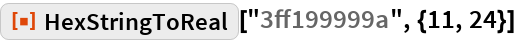Out=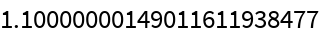## Requirements

Wolfram Language 11.3 (March 2018) or above